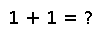# Dict

``````..  Dict([itr])

``Dict{K,V}()`` constructs a hash table with keys of type ``K`` and values of type ``V``.

Given a single iterable argument, constructs a :obj:`Dict` whose key-value pairs
are taken from 2-tuples ``(key,value)`` generated by the argument.

.. doctest::

julia> Dict([("A", 1), ("B", 2)])
Dict{ASCIIString,Int64} with 2 entries:
"B" => 2
"A" => 1

Alternatively, a sequence of pair arguments may be passed.

.. doctest::

julia> Dict("A"=>1, "B"=>2)
Dict{ASCIIString,Int64} with 2 entries:
"B" => 2
"A" => 1``````

## Examples

``````julia> dict = Dict()
Dict{Any,Any} with 0 entries
``````
``````julia> dict = Dict(["one"=> 1, "two"=> 2, "three"=> 3])
Dict{ASCIIString,Int64} with 3 entries:
"two"   => 2
"one"   => 1
"three" => 3``````

Checking you are not a robot: